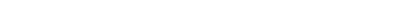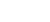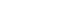Success on the GMAT depends on many skills, and one skill that can help a student make enormous progress is the skill of asking excellent questions.  In order to discuss this skill, first let’s look at a relatively straightforward practice problem.(A) 14

(B) 26

(C) 28

(D) 32

(E) 38

I will show a full solution at the bottom of this article.  Right now, let’s just suppose that you got this question wrong and wanted to ask about it.  The correct answer is the lowest of the five answer choices, so for example, if you went for the trap answer of 32, you fell right into one of the mistakes that the question was specifically designed to elicit.  Questions writers regular incorporate trap answers like big butterfly nets, and simply let hordes of test-takers run into them.  The more you, as a student, can recognize these traps, the more you can set yourself apart from the  mass of ordinary test takers.  I will discuss this more in the solutions below.

Suppose you did this questions, got it wrong, and didn’t have access to an immediate explanation for the question.  Suppose you wanted to get an explanation from an expert, either on a blog or on a forum such as GMAT Club.  How would you ask your question?

Here’s what many people might say:

2) I don’t get this.

I see questions of this ilk often.  These are abysmally poor questions.  If I were to assign a grade to such a question, I would give an F.  Asking a questions of this sort does not indicate any willingness, on the part of the student, to engage the material and put significant energy into the process of coming to a deeper understanding.

Notice, that questions like this require almost no effort to write.  One could write one of these questions and post it in under 15 seconds.  Right there, that’s an indication that it’s not necessarily going to help you.  You are not going learn the most and make the most progress unless you put a significant amount of effort into your own cause.

Another problem with these question is: think about it from the perspective of the expert who gets such a question.  I certainly understand how to solve this problem myself, but if  student tells me simply “I don’t understand. Please help,” then I don’t know where that student is stuck.  Does that student not know how to factor quadratics at all?  Did the student fall for the trap answer of (D)?  Did the student make a simple arithmetic mistake?  Does the student not understand algebra in the least?  Given that meager question, I have absolutely no idea what would best help the student.

The presumption of such a question is that the teacher, not the student is responsible for the process of education.  This is incorrect.  Education is not something a teacher or test prep company does to you.  Education is not a spectator sport.  Education is primarily something you do to yourself, by yourself, for yourself, and teachers or test prep companies simply provide the support and resources to enable you to educate yourself.

The art of asking excellent questions

The habits of excellence can set a student apart from others.  Excellence always takes more work, and many students avoid it for that very reason.  Many people don’t realize that, in choosing to exert a minimum of effort in many things related to their studies, they are implicitly choosing mediocrity.  The great Law of Mediocrity is: if you put in about the same effort as most people, then you probably will get about the same results as most people.  The corollary is: if you want results that stand out, you need to put in outstanding effort.  The habits of excellence require outstanding effort, and one of these habits is asking excellent questions.

Here are some examples of excellent questions:

4) When I looked at the problem, I saw right away that we could solve the first equation by subtracting 8 from both sides and solving x2 = 9 to get x = 3.  I didn’t know how to solve that other equation, so I am glad I didn’t have to deal with it.  From there, I simply plugged 3 into 3x + 23 to get 9 + 23 = 32.  I chose (D), but that does not agree with the OA.  What did I do wrong?

5) I began by moving the 8 to the other side, and then I solved x2 = 25 to get x = ±5.  I then factored the quadratic: x2 – 2x – 15 = (x + 3)(x – 5) = 0, x = –3 or x = +5, so because of the combined constraints of both equations, we see x must equal +5, and therefore 3x + 23 = 38, which would be (E), but that’s not the OA given.

Summary

One of the habits of excellence is asking excellent questions.  Many students don’t do this, and are mystified about why they continue to struggle and cannot retain or apply the valuable information they have learned.  I urge you to embrace the habit of asking excellent questions and all the habits of excellence.  If you have experiences with your own practice of asking excellent questions, we would love to hear from you in the comments section.

Practice problem explanation

1) In the first equation, we need to subtract 8 from both sides, which gives us:When we ourselves take a square root, we need to remember to include both the positive and the negative root.  See this GRE post for details.  We get two roots from this:From this equation alone, we cannot determine unique value of x.  We have to factor the second equation.The only root that is common to both equations is x = –3, so this must be the value of x.  This means

3x + 23 = 3(–3) + 23 = –9 + 23 = 23 – 9 = 14

•